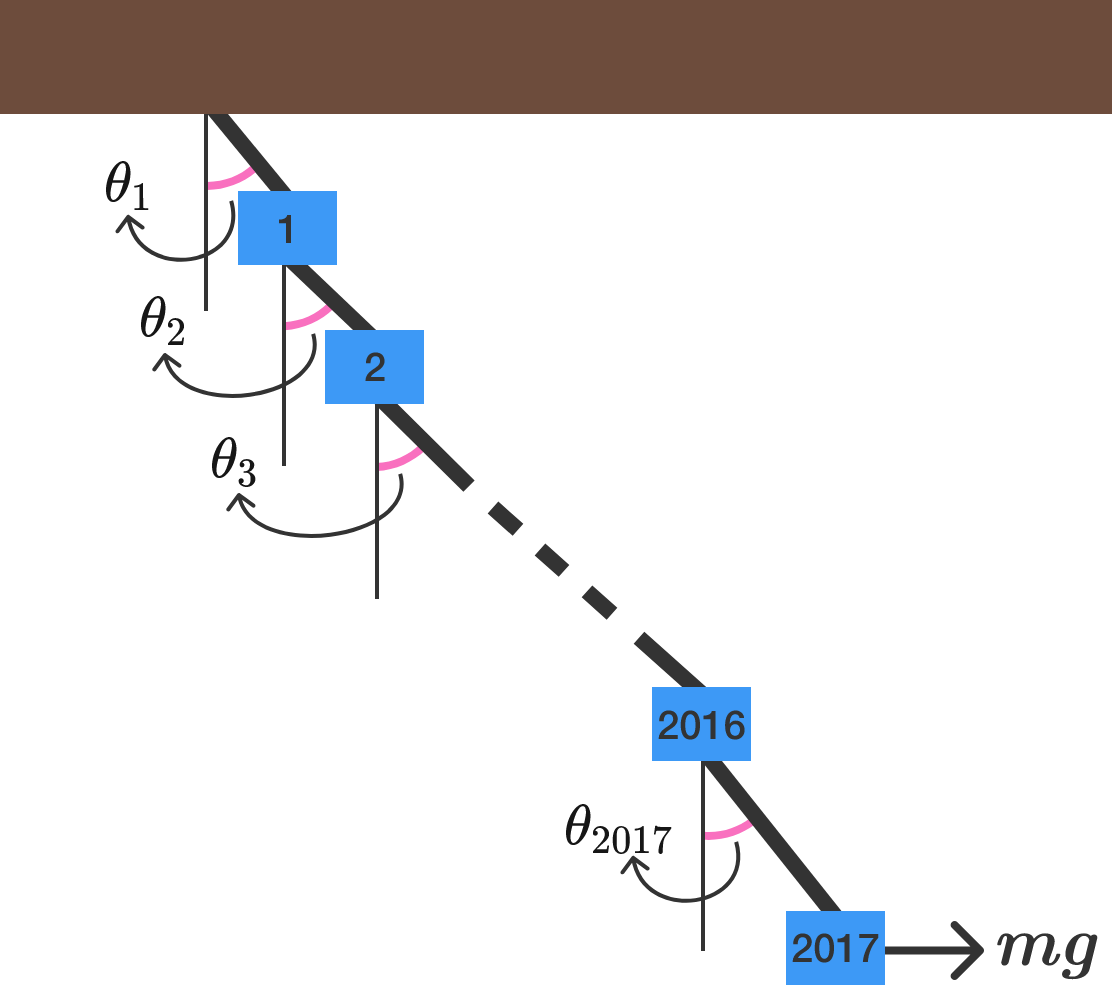2017 blocks2017 blocks each of mass $m = \SI{20.17}{\kilogram}$ are connected by massless strings and the whole system is fixed to a rigid ceiling, as shown above.

A constant force $F = mg$ acts on the $2017^\text{th}$ block and displaces it. As a result, the entire system is disturbed and subsequently settles into a stationary equilibrium. In the equilibrium position, the string above block $i$ subtends an acute angle of $\theta_i$ with the vertical.

What is $\theta_1$ (in degrees) to 3 decimal places? Take acceleration due to gravity as $g=\SI[per-mode=symbol]{9.80}{\meter \per \second \squared}.$

×